# Defining Centrifugal Loads

You can define a centrifugal load to a part or an assembly in static, frequency, buckling, or nonlinear studies.

To define centrifugal load to a model:

1. In the Simulation study tree, right-click the External Loads icon and select Centrifugal.
2. In the PropertyManager, select an axis, edge, or cylindrical face for direction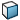.
3. Under Centrifugal Force, do the following:
1. Select the Units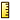for the angular velocity or acceleration.
2. Enter a value for the Angular Velocity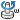. To reverse the direction of the angular velocity, select Reverse direction.
3. Enter a value for the Angular Acceleration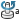. To reverse the direction of the angular acceleration, select Reverse direction.
4. In nonlinear studies, you can define time-dependent centrifugal forces by associating them with a time curve. Under Variation with Time select:
Option Description
Linear To use a default linear time curve which passes through the points (0,0) and (tend, A). Where A is the value of the angular velocity or angular acceleration specified in the Centrifugal Force box and tend is the End time specified on the Solution tab of the Nonlinear dialog box.
Curve To use a user-defined time curve. Click Edit to define or import a time curve. The angular velocity/angular acceleration is calculated at any time by multiplying the value specified above by the Y value of the time curve. Click View on the PropertyManager to display the actual time-dependent centrifugal force components.
5. Click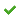.
The centrifugal load symbol is shown at the axis of rotation. The model spins about the specified axis.